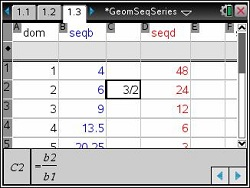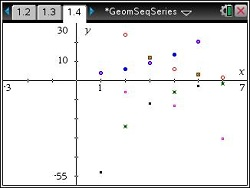# Activities

••• ##### Subject Area

• Math: Algebra II: Sequences
• Math: Algebra II: Series

• ##### Author9-12

45 Minutes

• ##### Device
•TI-Nspire™ CX/CX II
•TI-Nspire™ CX CAS/CX II CAS
• TI-Nspire™
• TI-Nspire™ CAS
• ##### Software

TI-Nspire™
TI-Nspire™ CAS

3.2

## Geometric Sequences & Series

#### Activity Overview

In this activity, students begin by finding common ratios of geometric sequences on a spreadsheet and then create scatter plots of the sequences to see how each curve is related to the value of the common ratio and/or the sign of the first term of the sequence. Students then generate sequences and develop a general explicit formula for the sequence. This is followed by discovering the geometric mean of two numbers and finding the sum of a geometric series.

#### Key Steps

•There are five finite sequences on page 1.3.  They appear in Columns B, D, F, H, and J.  Each has six terms.  Instruct students to find the ratio of each term and its previous term by writing and copying formulas. In cell C2, students need to type =b2/b1 to find the ratio between the first two terms.  The cell displays 3/2; but notice that when the cell is selected, the formula appears at the bottom of the screen.

•On page 1.4, students will create the scatter plots of the sequences.  They will make a conjecture about the common ratio, and/or the sign of the first term of the sequence in connection with the scatter plot.